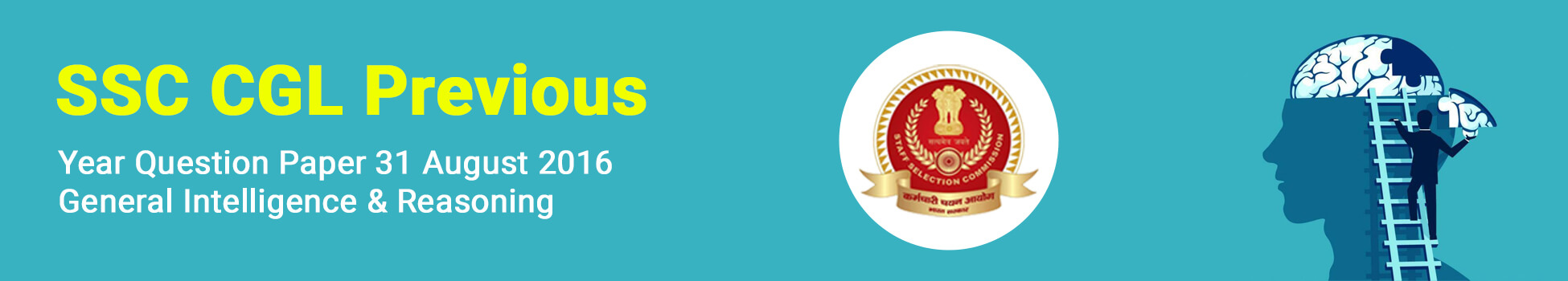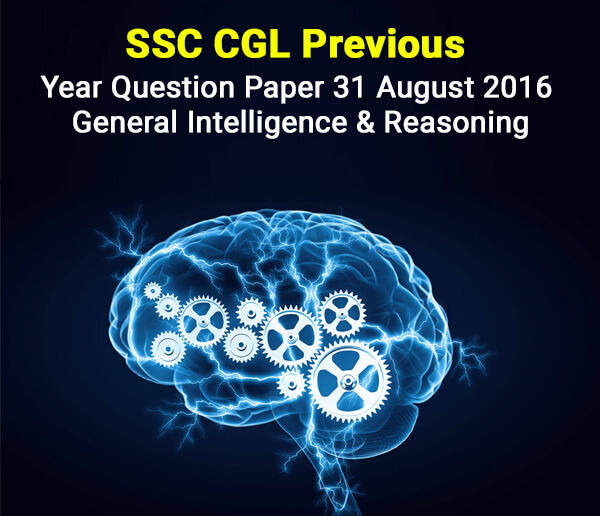SSC CGL Previous Year Question Paper 31 August 2016 - Genera - Study24x7# Welcome Back

or

By Registering, you agree to our and of use.

# SSC CGL Previous Year Question Paper 31 August 2016 - General Intelligence & Reasoning

Published on 15 February 2020Question 1.Select the related word/letters/numbers from the given alternatives:

CURE : DISEASE :: HEAL : ?

a. Illness

b. Injury

c. Recover

d. Sick

Question 2.Select the related word/letters/numbers from the given alternatives:

DBCE : QOPR :: JLKI : ?

a. YWXU

b. WYXV

c. WXYV

d. WYVX

Question 3.Select the related word/letters/numbers from the given alternatives:

6 : 42 :: 12 : ?

a. 48

b. 72

c. 60

d. 84

Question 4Find the odd word/letters/number pair from the given alternatives.

a. High-Up

b. Past-Present

c. Often-Seldom

d. Fresh-Stale

a. AOU

b. EOI

c. UIE

d. ALO

Question 6. Find the odd word/letters/number from the given alternatives.

a. 13

b. 17

c. 29

d. 87

Question 7.Arrange the following words as per order in the dictionary and choose the one that comes first:

1. Temple 2. Tenant 3.Terminate 4.Temperature

a. Temple

b. Tenant

c. Terminate

d. Temperature

Question 8.A series is given, with one term missing. Choose the correct alternative from the given ones that will complete the series.

NOM, QRP, TUS, ?

a. WAX

b. HUT

c. WXV

d. HTU

Question 9.A series is given, with one term missing. Choose the correct alternative from the given ones that will complete the series.

1,3,7,13,21,?

a. 27

b. 29

c. 31

d. 33

Question 10.If it is Saturday on 27thSeptember, what day will it be on 27thOctober of the same year?

a. Thursday

b. Sunday

c. Friday

d. Monday

Question 11.The ratio of the ages of man and his wife is 4:3. After 4 years, the ration will be 9:7. If at the time of marriage, the ratio was 5:3, how many years ago were they married?

a. 12

b. 24

c. 5

d. 8

Question 12.From the given words, select the word which cannot be formed using the letters of the given word.

ALTERNATIVES

a. ALTER

b. NATIVE

c. TEN

d. NATIONAL

Question 13.If code P is denoted by 7, X by 9, M by 5, Z by 8, L by 2, T by 1, then ZLTPXM will be

a. 812851

b. 821591

c. 812715

d. 821795

Question 14.If 24x2=84, and 32x3=69, then 13x3=?

a. 38

b. 93

c. 16

d. 10

Question 15.If + means division, - means multiplication, '÷' means subtraction, x means addition, and < means less than, then which of the following is false

a. (10+2)÷7 < (10÷7)+2

b. (10-7)x2 < (10x2)-7

c. (10X7)-2 < (10-2)x7

d. (10÷2)+7 < (10+7)x2

Question 16.Select the missing number from the given responses.a. 1

b. 4

c. 9

d. 25

Question 17.Ram went 20 meters to the north then turned towards east and walked another 5 meters, then he turned towards right and covered 20 meters. How far is he from the starting point?

a. 3 m

b. 4m

c. 5 m

d. 6 m

Question 18.One or two statements are given followed by two or more conclusions/assumptions, I, II, III, IV. You have to consider the statement to be true, even if it seems to be at variance from commonly known facts. You are to decide which of the given conclusions/assumptions can definitely be drawn from the given statement.

Statement:

1. All goats are tigers.

2. All tigers are lions.

Conclusion:

I. All tigers are goats.

II. All lions are tigers.

III. No goat is a lion.

IV. No lion is a goat.

a. Only Conclusion III & IV follow

b. Only Conclusion I & II follow

c. None of the Conclusions follows

d. All Conclusions follow

Question 19.In the question figure how many squares are there in all? Select from the given alternatives.a. 12

b. 14

c. 10

d. 11

SSC CGL tier 1 Exam : preparation strategy

Question 20.In the following figure, triangle represents teachers, square represents merchants and circle represents social workers. Which number space represents Teachers, who are social workers?a. 3

b. 2

c. 4

d. 5

Question 21.Which answer figure will complete the pattern in the question figure?a.b.c.d.Question 22.From the given answer figures, select the one in which the question figure is hidden/embedded.a.b.c.d.Question 23.A piece of paper is folded and cut as shown below in the question figures. From the given answer figures, indicate how it will appear when opened.a.b.c.d.Question 24.If a mirror is placed on the line MN, then which of the answer figures is the right image of the given figure?a.b.c.d.SSC CGL 2020 Syllabus - Exam Pattern

Question 25.In this question, the sets of numbers given in the alternatives are represented. The columns and rows of Matrix I are numbered from 0 to 4 and that of Matrix II are numbered from 5 to 9. A letter from these matrices can be represented first by its row and next by its column, e.g., D can be represented by 02, 10, etc., and 'R' can be represented by 55, 67, etc. Similarly you have to identify the set for the word 'BEAR'.a. 13,11,12,78

b. 31,22,23,97

c. 42,34,42,79

d. 23,50,33,67

All The Best To All The SSC CGL 2020 Aspirants !

Write a comment...